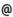## Babes-Bolyai University of Cluj-Napoca Faculty of Mathematics and Computer Science Study Cycle: Graduate SUBJECT

 Code Subject MI094 Neural Networks and Applications
 Section Semester Hours: C+S+L Category Type Computer Science - in Hungarian 8 2+1+1 optional Mathematics-Computer Science - in Hungarian 8 2+1+1 optional
 Teaching Staff in Charge
 Lect. CSATO Lehel, Ph.D.,  csatolcs.ubbcluj.ro
 Aims The course “Artificial neural network and applications” aims at familiarizing students with advanced concepts from modern artificial intelligence and basics of machine learning. The accent of the course is on studying adaptive methods based on neural networks and from the general family of machine learning algorithms. We aim at studying the general concept of a “learning machine” as well as their utility and usability for different problems and different algorithms with the emphasis on applications of the studied algorithms.
 Content • Machine Learning Models (weeks 1-2) ref. [1,2,9]: • Neural networks, adaptive models [1,9],(2 h.) • Neural network solutions: classification, regresssion [2,9].(2 h.) • The EM Algorithm (weeks 3-4) ref. [1,5]: • Clustering methods, problems, solutions [1,5],(2 h.) • The EM algorithm and applications [1,5],(2 h.) • Component-based analysis (weeks 5-8) ref. [1,3,5,9]: • Basis of stochastic modeling – matrices, eigenvalues [1,9],(2+2 h.) • Principal components for de-noising [3,5].(2 h.) • Principal components analysis .(2 h.) • Bayesian modeling (weeks 9-11) ref. [2,3,4,5]: • Introduction, different parameter estimation methods [2,3],(2 h.) • Hierarchic models and estimating parameters [2,5],(2 h.) • Bayesian models, using probabilities: a-posteriori and predictive entities [4,5].(2 h.) • Hidden Markov Models (HMMs) (weeks 12-14) ref. [6,7]: • HMMs, definitions, estimation methods ,(2 h.) • HMM applications (1) sound recognition [6,7],(2 h.) • HMM applications (2) gene sequencing [6,7],(2 h.)
 References . Bishop C.M (2006) Pattern Recognition and Machine Learning, Springer Verlag. . Russell S, Norvig P. (2003) Artificial Intelligence: A Modern Approach (Second Edition), Prentice Hall. . Mitchell T (1997) Machine Learning, McGraw Hill. . Bernardo J.M, Smith A.F.M (2000) Bayesian Theory, John Wiley & Sons. . MacKay D.J.C (2003) Information Theory, Inference and Learning Algorithms, Cambridge University Press, HTTP: http://wol.ra.phy.cam.ac.uk/mackay/itila/book.html. . Rabiner L.R, Juang, B.H (1986) An introduction to Hidden Markov models, IEEE ASSP Magazine, pp: 4-15. . Durbin R, Eddy S.R, Krogh A, Mitchison G (1999) Biological Sequence Analysis: Probabilistic Models of Proteins and Nucleic Acids. Cambridge University Press. . Hyvärinen A, Karhunen J, Oja E (2001) Independent Component Analysis, Wiley-Interscience. . Barto A. (2002): Statistical Pattern Recognition, John Wiley & Sons.
 Assessment The exam note comprises of two parts: a first – 40% - is the evaluation of individual or group work in seminar and practical sessions in the laboratories. A second part of 60% is the examination in the exam period at the end of the semester. Only the students with all practical problems solved will be admitted for the final exam.
 Links: Syllabus for all subjects Romanian version for this subject Rtf format for this subject